﻿ Bellevue

# Bellevue

The Bellevue codec is an experimental image compression codec. It takes concepts of transformation codecs and of subband coding, but based on a very simple algorithm. Unfortunately it is not very effective at the current state, but i publish it for demonstration. It allowed me to understand how compressors work. An programming just with BBedit, Terminal and Preview is fun.

#### The Bellevue transformation

Subband coding separates the image signal by frequency. It uses filters to create a low-band and a high-band version of the signal. For images, this is done normally once vertically and once horizontally independently. We then have four subimages: low-low, low-high, high-low and high-high. The low-low subimage has the main energy, while the subimages with high components are more or less medium gray. The high-band subimages can then be quantized reducing the file size.

The Bellevue transformation creates also four subimages, but based on a transformation of a square of two times two pixels. We will name the four pixels of the uncompressed image a,b,c,d and the four pixels of the transformation m,x,y,t

 a b c d
 m x y t

The following transformation is reversible:

```m = (a + b + c + d) / 4;  // the mean value of the four pixels
x = (a - b + c - d) / 4;  // the horizontal contrast
y  = (a + b - c - d) / 4;   // the vertical contrast
t = (a - b + c - d) / 4;   // the diagonal contrast
a = m + x + y + t;
b = m - x + y -t;
c = m + x - y - t;
d = m - x - y + t;```

The transformation is very simple and consists mainly of additions and substractions only. It therefore can be very fast.

With this transformation we create four subimages. The m is lowband and contains the main energy, and x,y,t are the highband subimages.

#### Implementation of Bellevue transform in 8-bit resolution

The Bellevue transformation is lossless and completely reversible in floating point tnumbers, but for images we have to deal with 8bit resolution. The minimum value is therefore 0 and the maximum value 255.
The transformation for m creates quarters of integers, so we have to round. We add 2 and use the bitshift operator for the division. So the medium error for m is 0.5

`m = (a + b + c + d + 2) >> 2;`

The transformation for x,y,z,t creates value between -128 and 127, so we add 512+2 and use the bitshift operator for the division. The medium error for y,y,t is 0.5.

```x = (a - b + c - d + 514) >> 2;
y = (a + b - c - d + 514) >> 2;
t = (a - b + c -d + 514) >> 2;```

And we have to compensate for the shift on the inverse transformation. Note that a has a different constant, as all x,y,t are positive.

```a = m + x + y + t - 384;
b = m - x + y -t + 128;
c = m + x - y - t + 128;
d = m - x - y + t +128;```

#### The Bellevue transformation on the sample image

The Bellevue transformation creates an image of equal size, so we can inspect the transformation of the image. We will use the standard Lena image, which is generally used to test image compressors. Further tests on other test images show that the results are consistent. On the left, the original Lena image, on the right, the Lena image with the Bellevue transform. For the webpage, the images have been exported as lossless PNG images.

`./bellevue -l 1 -z 0 -q 1 -i -r lena.tga lena0.tga `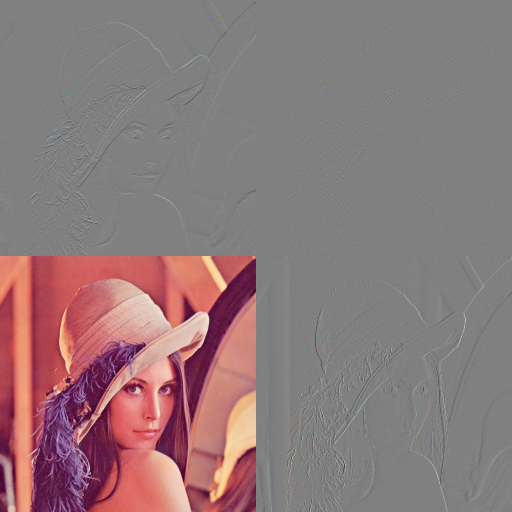We decompress the image and compare the result with the original image. The PSNR is 55.09 dB and the the SSIM is 0.997.

```./bellevue -d lena0.tga lena1.tga
./psnr lena.tga lena1.tga
PSNR = 55.09 dB 0.997```

16% of the byte values are now 128. Our goal of the compression is to get as many byte values as possible at 128 and concentrate the energy to the remaining pixels we will compress using standard tools.

The transformation can be made on more than one level. In this case, we take the lowband subimage and reapply the transformation to the it. Below the Bellevue transformation is done twice and three times

```./bellevue -l 2 -z 0 -q 1 -i -r lena.tga lena2.tga
./bellevue -l 3 -z 0 -q 1 -i -r lena.tga lena3.tga ```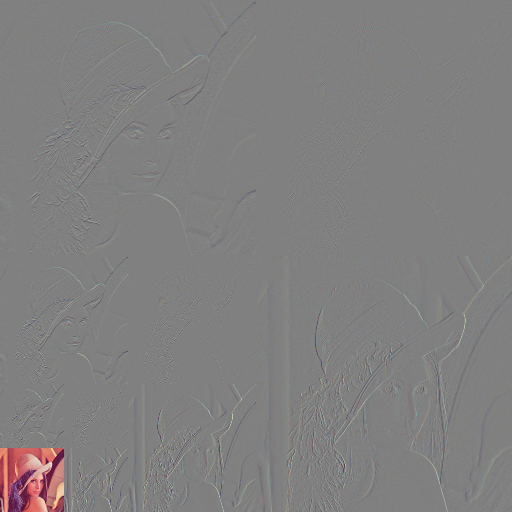We have now 21% and 22% of byte vales at 128, but the returns are diminishing. At this image size, further transformation will not make sense. We must take also into account that the rounding errors create noise and reduce the PSNR and the SSIM

```./bellevue -d lena2.tga lena2a.tga
./psnr lena.tga lena2a.tga
PSNR = 51.75 dB 0.994
./bellevue -d lena3.tga lena3a.tga
./psnr lena.tga lena3a.tga
PSNR = 49.67 dB 0.992```

#### Quantization

The quantization is the main lossy component in the compression. It defines a resolution of the values and rounds to the next integer. The goal is to get less variation of the byte values.

The Bellevue compressor quantizes only the x,y,t values. We provide an integer division by the factor q. As the values are shifted by 128, we need to substract by the pivot 128 first, add q/2 to round properly and then make an integer division by q. Finally we add the pivot 128 again.

`quantize(x,q,pivot) ( x>pivot ? (((x-pivot+q/2) / q ) + pivot ) :  (((x-pivot-q/2) / q ) + pivot ) )`

Some quantizers immediately remultiply by the quantizer to get the original dimension of the value. We won't do that. For a later stage, it is an advantage for us to keep the values as near as possible at 128. At level 1, the medium error for a quantizer q is q/2.

If there is muliple levels of transformation, the quantization is applied on each level, but each lower level at half the value. We do this because the errors at lower levels concern more pixels.

Below is the Bellevue transformation at level 2 and q=4 and q=16. At q=4, 44% of the byte values are 128, at q=16, 86% are 128.

```./bellevue -l 2 -z 0 -q 4 -i -r lena.tga lena4.tga
./bellevue -l 2 -z 0 -q 16 -i -r lena.tga lena5.tga ```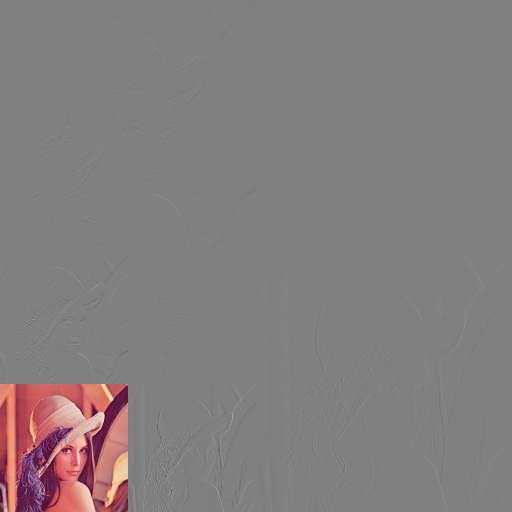And the reconstruction

```./bellevue -d lena4.tga lena4a.tga
./psnr lena.tga lena4a.tga
PSNR = 42.67 dB 0.956
./bellevue -d lena5.tga lena5a.tga
./psnr lena.tga lena5a.tga
PSNR = 35.46 dB 0.788```As we can see, the quantization degrades the image and introduces at some higher q a block artefact. This happens because x,y,t becoming 128 creates a block of 4 identical pixels.

#### Blockfilter

The blockfilter uses the fact that the x and y values are correlated from block to block. x and y are part of a low band contrast between the neighbouring blocks. Therefore we use a prediction. We predict that x is determinated by m of the left block and m of the right block and retain only the difference between the actual x and the prediction.
The distance between the mean values is four pixels. As the pixels are influenced both by horizontal contrast, we go conservative and divide by eight.

 al bl a b ar br cl dl c d cr dr
``` ml =  (al + bl + cl + dl + 2) >> 2;   // mean of left block
mr =  (ar + br + cr + dr + 2) >> 2;   // mean of right block
mt =  (at + bt + ct + dt + 2) >> 2;   // mean of top block
mb =  (ab + bb + cb + db + 2) >> 2;  // mean of the bottom block
x -= (ml - mr ) >> 3;
y-= (mt - mb ) >> 3;```

The blockfilter has two effects: With high q, the default byte value 128 results not in a block, but in bilinear interpolation. And we have still more 128 values. In the algorithm we know already ml and mt, but we have to calculate mr and mb.

Below is the Bellevue transformation with blockfilter at level 2 and q=4 and q=16. At q=4, 44% of the byte values are 128, at q=16, 86% are 128.

```./bellevue -l 2 -z 0 -q 4 -i -r -b lena.tga lena6.tga
./bellevue -l 2 -z 0 -q 16 -i -r -b lena.tga lena7.tga ```And the reconstruction

```./bellevue -d lena6.tga lena6a.tga
./psnr lena.tga lena6a.tga
PSNR = 41.28 dB 0.938
./bellevue -d lena7.tga lena7a.tga
./psnr lena.tga lena7a.tga
PSNR = 35.96 dB 0.808```The blockfilter raises the 128 values at 46% for q=4 and 88% for q=16. The PSNR values are not better as the absolute errors are the same or higher due to rounding, but SSIM is better for q=16 and the subjective result removes the blocks so we will retain the blockfilter. The blockfilter adds a medium error of 0.5

The images below show the degradation with and without blockfilter

-l 2 -q 0..40-l 2 -q 0..40 -b#### Amplitude separation

When we look at the compressed image, we see that many byte values are near 128, but not completely. We want however as many sequantial values 128 as possible. We now procede at amplitude separation. For each byte value, we separate the high hex and the low hex. For each value between 128 and 143, the high hex would be 8. Most of the values are between 128 and 136, so we add a bias of 8 before we separate. This has two advantages: Most values will have the high hex at 8 and the low hex for the original 8 is 8, so most combined values will be 136.

This step is lossless, but it raises the default byte values of (now) 136 to 60% for q=4 and to 90% for q=16. In the image we see above the high hex, which is quite completely uniform and below the low hex, which is also quite uniform for q=16.

```./bellevue -l 2 -z 0 -q 4 -i -r -b -a lena.tga lena8.tga
./bellevue -l 2 -z 0 -q 16 -i -r -b -a lena.tga lena9.tga ```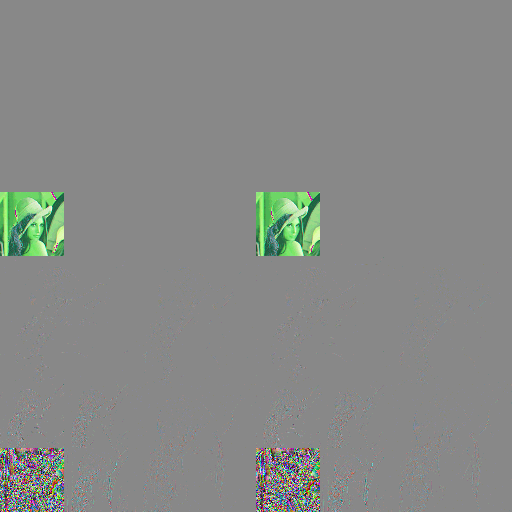#### Zero run length encoding

We use now a run length encoding, but a special variant. As 60-90% of the bytes are 136, we encode the run length only for this value. The decoding algorithm (easiser to describe) is like the following.

```value = *data++;
if (value == 136)
{
count = *data++;
for(i=0;i < count;i++) *buffer++ =value;
}
else
*buffer++ = value;```

This step is lossless. The black part of the image below shows the compression.
For low values of q it can happen that this step makes the data bigger. In this case, the zero run length encoding is not used.

```./bellevue -l 2 -z 0 -q 4 -i -r -b -a -Z lena.tga lena10.tga
./bellevue -l 2 -z 0 -q 16 -i -r -b -a -Z lena.tga lena11.tga ```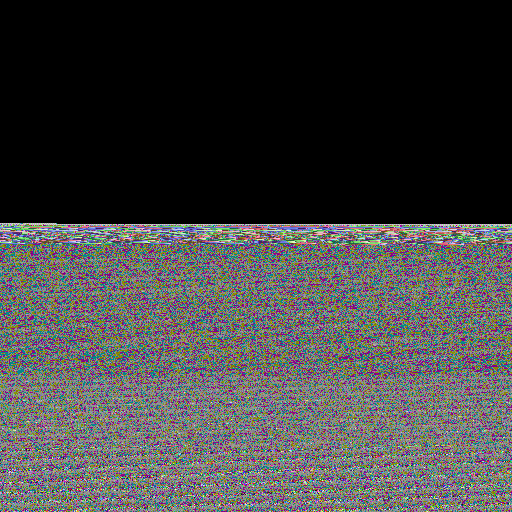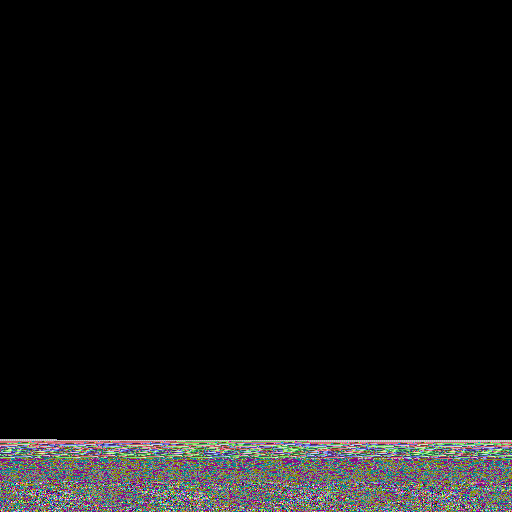As far as here, you can look at the compressed image like a regular TGA image. However the data is not yet compressed.

#### Deflate

As last step, we use deflate from zlib compression. The deflate has 9 levels, but at this stage, levels more than 3 only make the compression slower without reducing the file a lot. The resulting file cannot be viewed any more as a normal TGA file.

```./bellevue -l 2 -z 0 -q 4 -i -r -b -a -Z -z 1 lena.tga lena12.tga
...
./bellevue -l 2 -z 0 -q 16 -i -r -b -a -Z -z 9 lena.tga lena13.tga ```
 z 1 2 3 4 5 6 7 8 9 q=4 33.3% 32.9% 32.1% 31.8% 31.3% 30.9% 30.8% 30.6% 30.6% q=16 9.4% 9.3% 9.2 % 9.1% 9.0% 9.0% 9.0% 9.0% 9.0%

The Bellevue codec adds additional steps which allow all to gain some bytes.

YUV color space: The RGB image is transformed into YUV space because there is a lot of correlation between R, G and B. Taking the original image transformation, we get more 128 values.
Planar mode: The bytes for each channel are ordered separately, because they are more correlated than the values between the channels. These does not result in more 128 values but more 128 neighbours.

```./bellevue -l 1 -z 0 -q 1 -i  lena.tga lena14.tga
./bellevue -l 1 -z 0 -q 1 lena.tga lena15.tga ```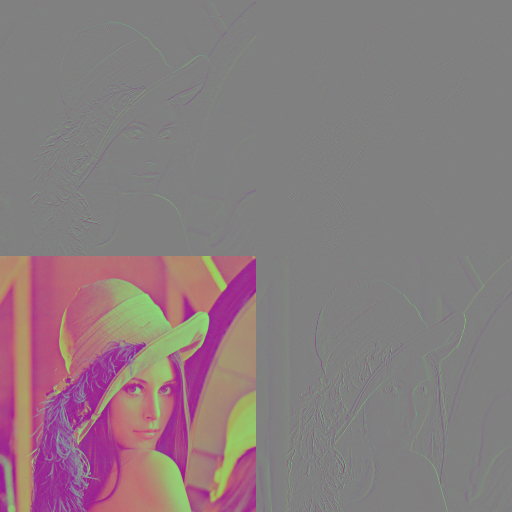RGB interleaved RGB planar RGB planar YUV planar q=4 33.3% 34.0% 27.7 % 27.3 % q=16 9.4% 10.6% 7.1% 6.8%

The planar mode does only reduce the data with YUV, not RGB.

Color subsampling''': The chroma can be subsampled which makes sense if the image is originally 4:2:2. This reduction is lossy and only effective at lower q values. x is anyway 128 for higher q values for most blocks.

 YUV planar YUV planar -s q=4 27.3 % 22.3% q=16 6.8% 6.8%

#### File format

The Bellevue codec uses the TGA format because it is very simple. It has a small header, allows for space to store the compression parameters in identdata and is then followed by the data.
The compression parameters allow the program to retrieve the values at decompression.

The TGA by default has the first pixel line in bottom and not in top. For this reason the low subimage is on the bottom left and not on the top left.

#### Performance

We tested the performance of Bellevue against JPEG and JPEG2000 for a suite of known standard test images: Lena, Lena in black and white, Peppers, Barbara, Kodim19, Kodim23. We added two HD images: Carole (from a DCP master), Runners (iPhone original).

The parameters for Bellevue were

`./bellevue -l \$level -q \$q -z 1 -Z -a -b lena.tga lena2.tga`

And we used sips for the JPEG and the JPEG2000

```sips -s format jpeg -s formatOptions \$q lena.tga --out lena2.jpg
sips -s format jp2 -s formatOptions \$q lena.tga --out lena2.jp2```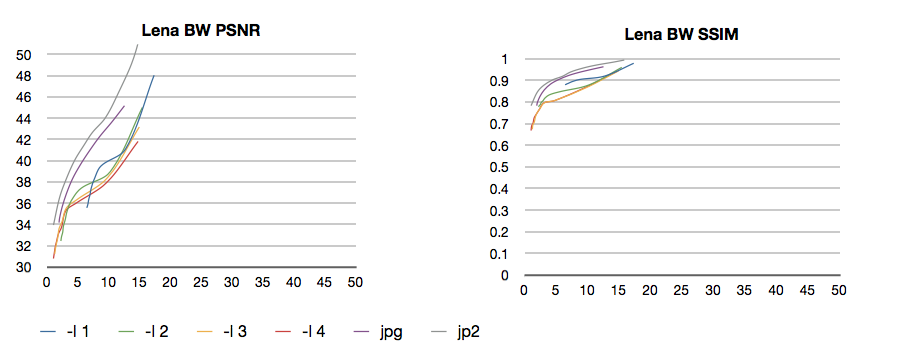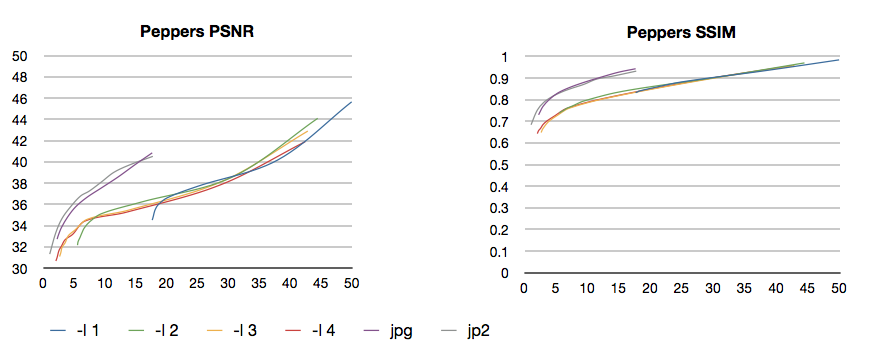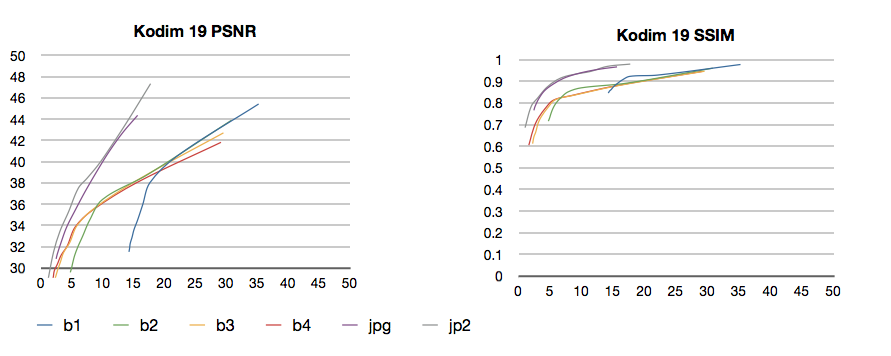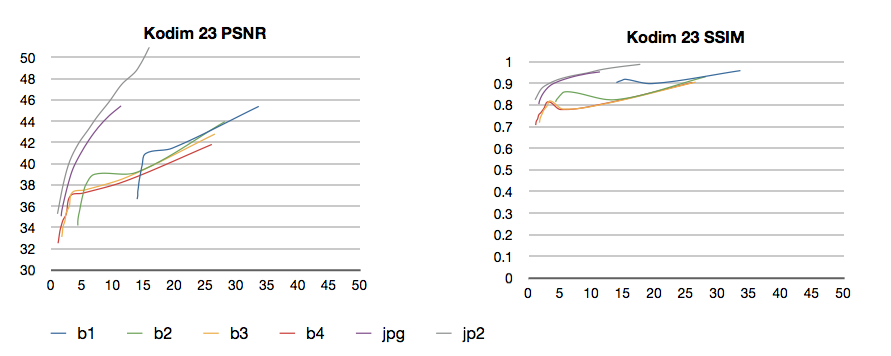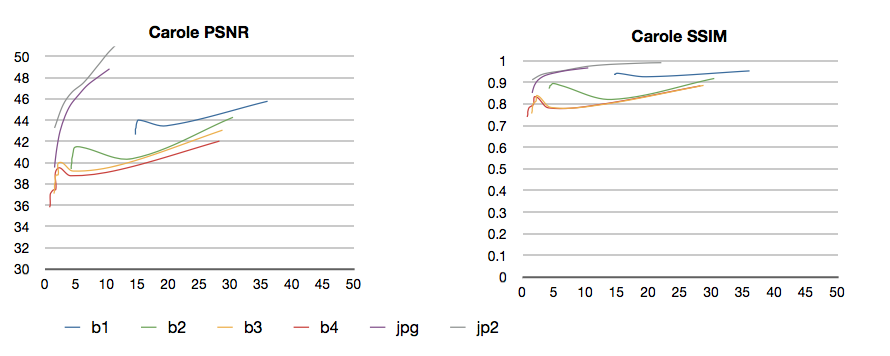As we can see, the PSNR for the Bellevue codec is always 3-4 dB below the JPEG and JPEG2000 for the same amount of compression. The black and white Lena performs better, so probably JPEG and JEPG2000 work also with color subsampling, which is not visible in the numeric results of PSNR and SSIM which are based only on Y and not on U and V. We see also that -b has a negative impact on low q, because without -b, the error is always lower and the PSNR always better for higher datarate. We see also that the optimal result is given with -l 2 at higher (5% +) and -l 3 at lower datarates. There is no difference between -l 3 and -l 4.

http://www.belle-nuit.com/site/files/source-bellevue.zip contains the complete source code in C++

main.ccp includes

• tga.php
• bellevue.cpp
• zlib.h and the precompiled libz.a (based on zlib-1-1.2.7)

mainpsnr.cpp is the utility to caculate PSNR and SSIM from two TGA images and includes

• tga.cpp
• psnr.cpp

http://www.belle-nuit.com/site/files/testimages.zip contains the test images.

The source code separates each step of compression to make it more clear. Everytime, the data is transformed to the buffer and the result written back to data. The sfxBellevue class provides the methods for the steps, they are executed in the main file. A real world codec could be faster and using less memory. The code is probably not very memory safe, but as the lifetime of the application is only one image, this is not a problem. As a library it probably would be.

#### Compiling

On MacOS 10.7 or 10.8:

```c++ libz.a main.cpp -o bellevue -O2
c++ mainpsnr.cpp -o psnr -O2```

Matthias Bürcher 5.1.2013